# House Price Prediction

An in-depth comparison of statistical approaches and advanced machine learning techniques

## 1. Introduction:

Imagine you have decided to take the plunge and put your beloved home on the market. A pressing question immediately presents itself: what is your house truly worth? This is a critical question that homeowners grapple with. An accurate estimation could be the difference between maximizing profit or underselling your most valuable asset. Even worse, if the price is set unreasonably high, potential buyers may be scared off, resulting in the property remaining unsold. This seemingly simple question of house valuation is complex and multifaceted, as it requires an understanding of various elements that could significantly impact a house's price.

This is a regression problem. Regression is a statistical methodology used to examine the relationship between one dependent variable (the variable we want to predict) and one or more independent variables (the variables used to make the prediction). It estimates how a unit change in the independent variables influences the dependent variable. Regression models are extensively used in various fields such as economics, finance, biology, and machine learning for predictive analysis. In the context of this problem, regression techniques are employed to predict house prices (the dependent variable) based on various housing features (the independent variables).

## 2. Data:

We are going to use the Ames Housing dataset to solve this problem. The Ames Housing dataset is a robust and rich collection of information about residential homes in Ames, Iowa. This dataset is highly regarded for its depth and comprehensiveness, making it a staple in predictive modeling and machine learning, particularly for regression tasks. It consists of 2930 observations. Each observation corresponds to a residential home, described through 80 variables including the sale price. However, we are going to a use simplified version of that dataset with 15 variables in this tutorial.

Columns and their explanations are listed below.

• sale_price: the property's sale price in dollars (dependent variable)
• lot_area: Lot size in square feet
• neighborhood: Physical locations within Ames city limits
• overall_quality: Overall material and finish quality
• overall_condition: Overall condition rating
• year_built: Original construction date
• basement_area: Total square feet of basement area
• first_floor_area: First Floor square feet
• second_floor_area: Second floor square feet
• full_bath: Full bathrooms above grade
• half_bath: Half baths above grade
• bedrooms: Number of bedrooms above basement level
• kitchens: Number of kitchens
• garage_area: Size of garage in square feet
• pool_area: Pool area in square feet

Dataset overview can be seen using Octai's Import module.

## 3. Evaluation

In the context of house pricing, inaccurate predictions can lead to financial loss. If the predicted price is lower than the actual worth of the house, potential profits are forfeited. Conversely, if the predicted price overshoots the actual value, the house may fail to sell due to its perceived overvaluation. Therefore, the magnitude of the deviation from the actual price, whether an overestimation or an underestimation, can adversely affect profitability. Metrics such as Mean Absolute Percentage Error (MAPE) or Mean Absolute Error (MAE) are valuable in assessing the accuracy of these price predictions. We are going to use MAPE metric for evaluating our predictions.

Mean Absolute Percentage Error (MAPE) is a statistical measure used to understand the accuracy of a predictive model. It calculates the average of the absolute percentage differences between the actual values and their corresponding predicted values.

In simpler terms, it tells you, on average, by what percentage your predictions are off from the actual values. Lower MAPE values indicate a better fit of the predictive model to the data, meaning your predictions are closer to the actual values and are therefore more accurate. This metric is particularly useful in contexts where relative errors matter more than absolute errors, like in our house pricing scenario.

The formula for Mean Absolute Percentage Error (MAPE) is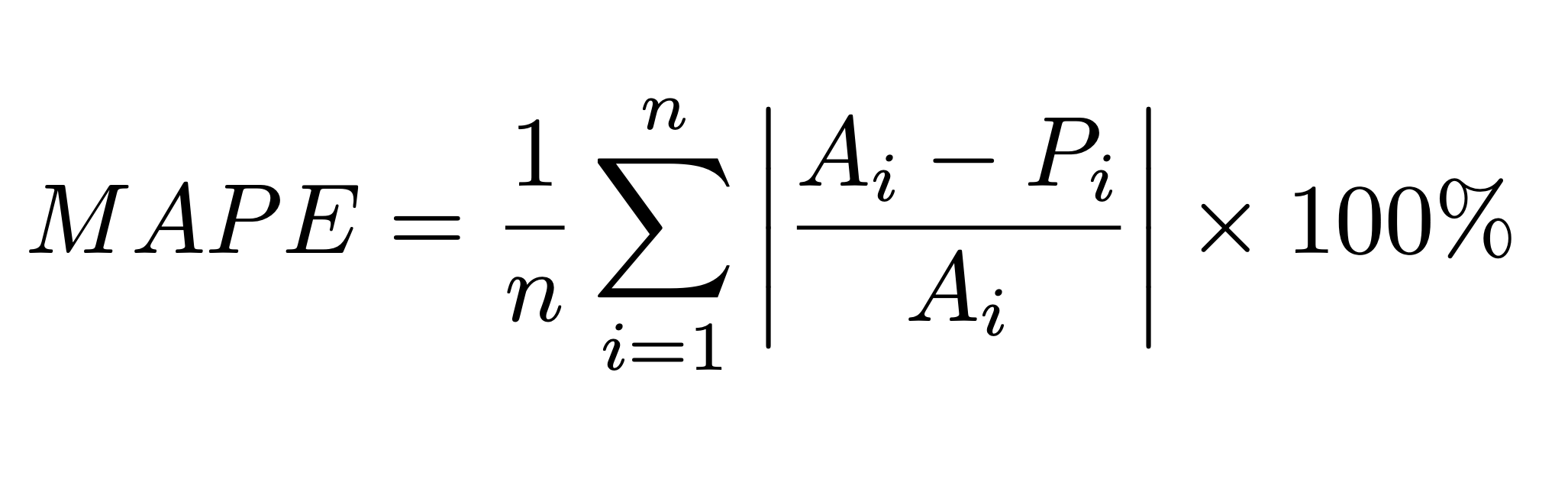## 4. Baseline Solution

### 4.1. Sale Price Median

Essentially, this code block is a simple "model" where the predicted sale price for every house is just the median of all house prices in the dataset. This isn't a sophisticated prediction - it's assuming that the sale price for any given house is just the middle-most value from all house prices. This simple model has a 32.05% mean absolute percentage error.

``````df['sale_price_median'] = df['sale_price'].median()
mean_absolute_percentage_error(df['sale_price'], df['sale_price_median'])
>>> 32.05
``````

All the predicted prices (`sale_price_median`) are set to the median value (160,000 in this case), and all of them appear as a horizontal line on this scatter plot at the y = 160,000 level.

This plot essentially helps in understanding how a simple median prediction model performs and sets a baseline for further, more complex models. It also visually emphasizes the need for a more sophisticated model that can account for the variability in house prices rather than predicting a constant value.

### 4.2. Sale Price Neighborhood Median

This code snippet is a slightly more advanced version of the previous one. This operation implies that we're making a prediction of a house's sale price based on the median sale price of other houses in the same neighborhood. It's a simple model assuming that houses in the same neighborhood have similar prices. This model has a 20.29% mean absolute percentage error.

``````df['sale_price_neighborhood_median'] = df.groupby(['neighborhood'])['sale_price'].transform('median')
mean_absolute_percentage_error(df['sale_price'], df['sale_price_neighborhood_median'])
>>> 20.29
``````

In this model, the predicted prices are set to the median value of each neighborhood. This means that houses in the same neighborhood have the same predicted price, causing a more linear form in the scatter plot. While this model is a significant improvement over the regular median model, it's still quite simple.

### 4.3. Sale Price Neighborhood Bedrooms Median

This suggests that we're predicting a house's sale price based on the median sale price of other houses in the same neighborhood and with the same number of bedrooms. This slightly advanced model assumes that houses within the same neighborhood and having the same number of bedrooms will have similar prices. This model has a 17.86% mean absolute percentage error.

``````df['sale_price_neighborhood_bedrooms_median'] = df.groupby(['neighborhood', 'bedrooms'])['sale_price'].transform('median')
mean_absolute_percentage_error(df['sale_price'], df['prediction'])
>>> 17.86
``````

In this predictive model, the expected house prices are determined based on the median value within each respective neighborhood and bedroom. As a result, houses within the same vicinity and the same number of bedrooms are assigned the same predicted price. This results in a more linear trend when visualized in a scatter plot.

The Mean Absolute Percentage Error (MAPE) of 17.86% from this model indicates that, on average, the predicted house prices deviate from the actual house prices by about 17.86%.

In other words, if you were to use this model to predict the price of a house, you could expect your prediction to be off by about 17.86% from the true price. For example, if a house's true price was \$100,000, on average, this model's prediction might be \$17,860 above or below this price.

Remember, this is an average measure. Some individual predictions might be much closer to the true prices, while others might be much farther off. It's also important to note that lower MAPE values indicate a better fit of the predictive model to the actual data, so a MAPE of 17.86% suggests there may be room for model improvement.

The predictions we made earlier can be created easily with Lambda nodes on Octai, as shown below.

## 5. Machine Learning Solution

Machine Learning (ML) can offer several significant advantages over baseline solutions, especially when dealing with complex datasets with multiple features, like housing data.

We are going to use LightGBM model here. LightGBM is a gradient-boosting framework that uses tree-based learning algorithms and is known for its high speed and performance.

### 5.1. Categorical Encoding

We are encoding our categorical columns here. Categorical encoding is a process of converting categories to numbers. Machine Learning algorithms work with numerical data, so you need to convert categorical data into numbers before you can use it for model training.

``````from sklearn.preprocessing import LabelEncoder

categorical_columns = ['neighborhood']
for column in categorical_columns:
df[column] = LabelEncoder().fit_transform(df[column])
``````

Same operation can be done easily by attaching the input column (`neighborhood`) to an Encoder node on Octai.

### 5.2. Train-Test Split

This code creates train-test split of your data, which is a crucial step in evaluating the performance of Machine Learning models. Here's why it's important:

Train-Test Split: In machine learning, we need a way to check how well our model will generalize to new, unseen data. One common method is to split our dataset into two parts: a training set and a test set.

Training Set: The model is trained on this data, which allows it to learn the relationships between the features (independent variables) and the target (dependent variable).

Test Set: This data is held out and not shown to the model during training. After the model is trained, it makes predictions on the test set. Since we know the actual outcomes for the test set, we can compare them to the model's predictions to evaluate how well the model is likely to perform on unseen data.

``````from sklearn.model_selection import train_test_split

df_train, df_test = train_test_split(df, test_size=0.2, random_state=42)
``````

### 5.3. Training and Validation

This piece of code is training a LightGBM model to predict house prices and then evaluating the model's performance using the Mean Absolute Percentage Error (MAPE).

``````from lightgbm import LGBMRegressor

model = LGBMRegressor(
num_leaves=32,
subsample=0.9,
subsample_freq=1,
min_data_in_leaf=20,
n_estimators=150,
objective='l1'
)

features = [
'neighborhood_encoded', 'bedrooms', 'kitchens', 'full_bath', 'half_bath',
'lot_area', 'basement_area', 'first_floor_area', 'second_floor_area', 'garage_area', 'pool_area',
'year_built', 'overall_quality', 'overall_condition'
]
target = 'sale_price'

model.fit(X=df_train[features], y=df_train[target])
df_test['lightgbm_prediction'] = model.predict(df_test[features])
mean_absolute_percentage_error(df_test['sale_price'], df_test['lightgbm_prediction'])
>>> 8.4
``````

This model has 8.4% mean absolute percentage error. It's two times better than the previous models. For comparison, if a house's true price is \$100,000, on average, this model's prediction might be \$8,400 above or below this price. The average error was \$17,860 for the same house using previous baseline model. In this case, using machine learning over baseline models can save \$9460 on average for a \$100,000 house.

Even though the code version looks easy, setting up a pipeline like this requires deep knowledge of machine learning and data science. Octai comes to help at this point. Octai's Model module is simple and intuitive for every level of machine learning practitioners.

After selecting a dataset, label (target column) and task type, a machine learning experiment can be started by pressing "Train" button.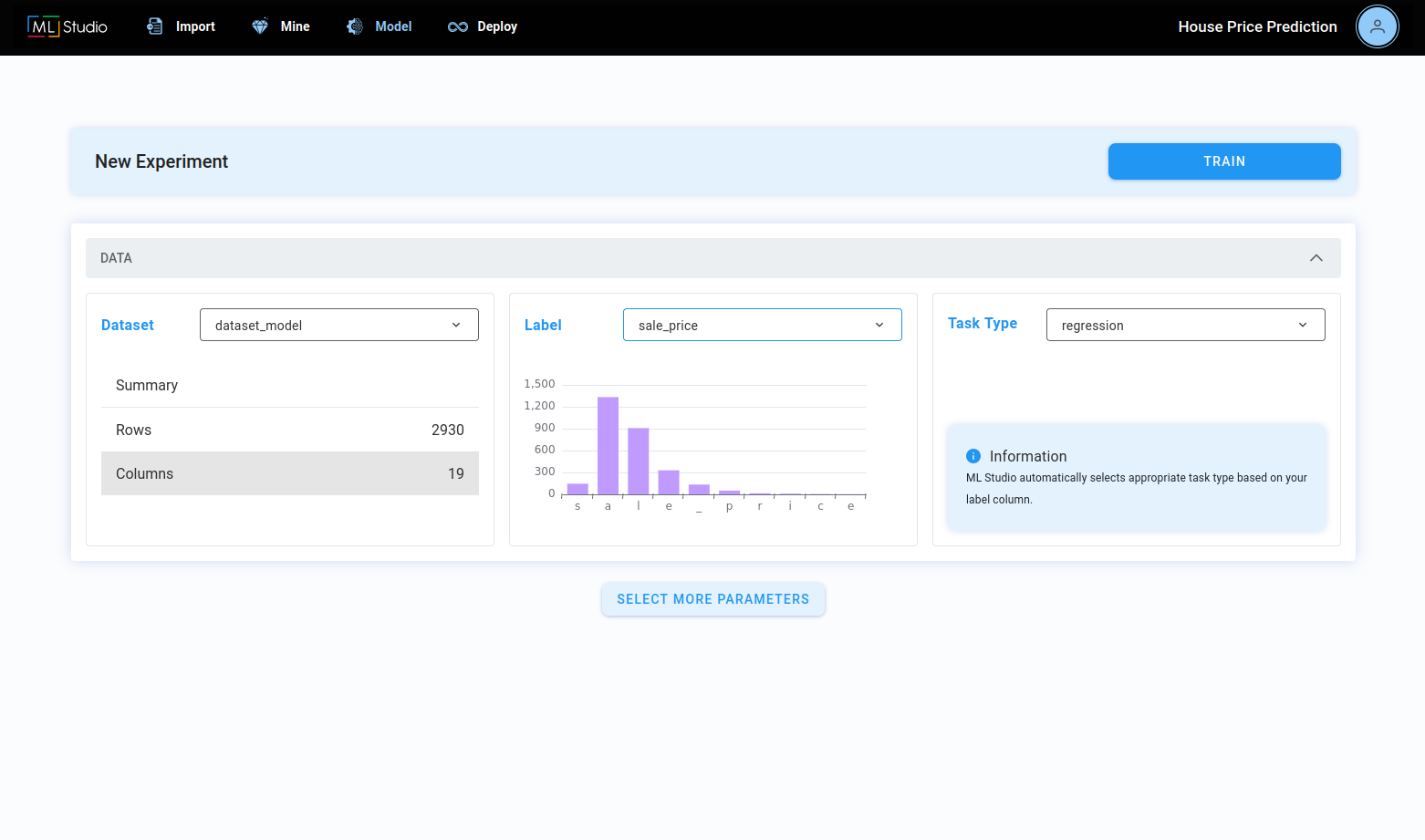However, since we created additional columns before, we have to select features that we are going to use in our model.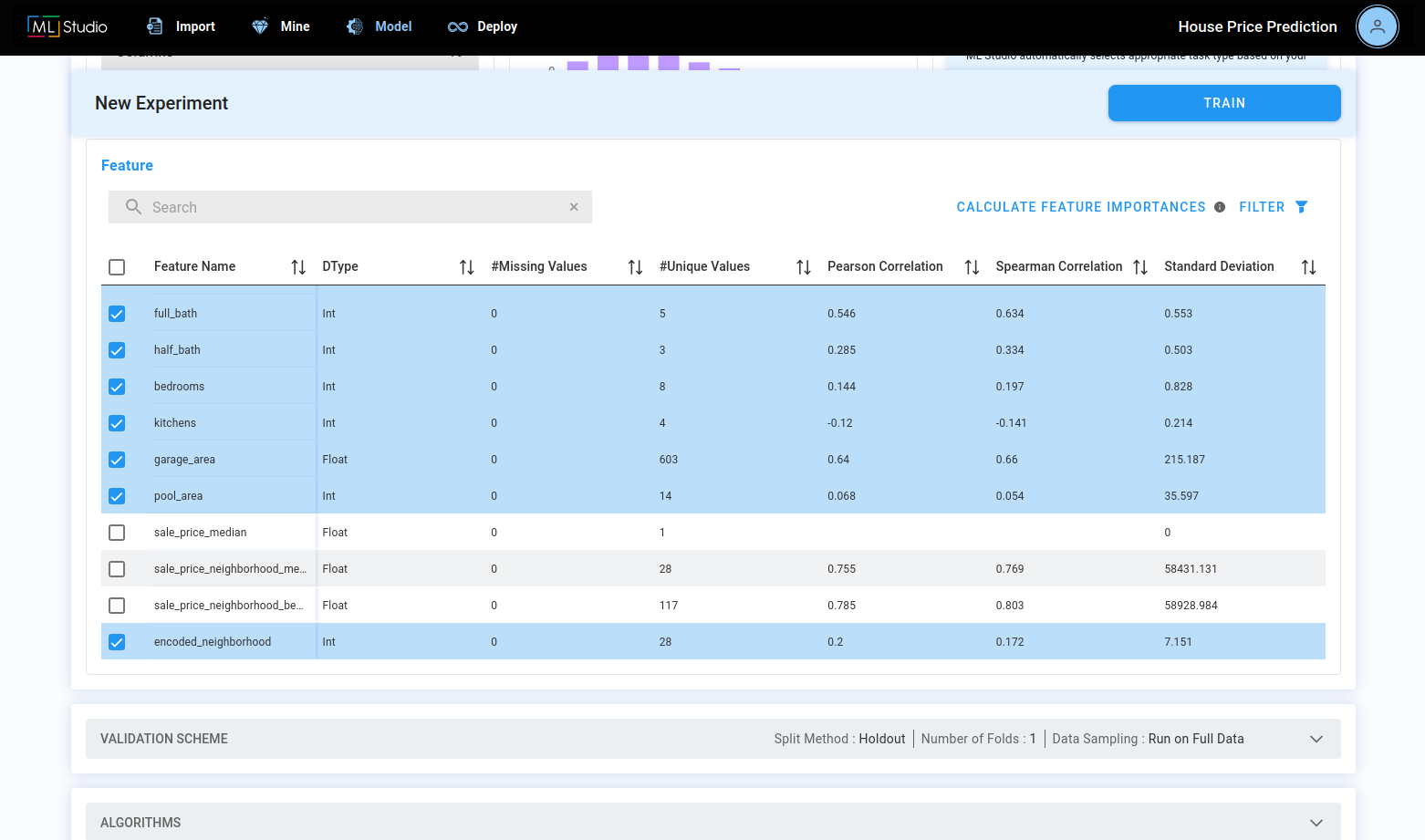After selecting the features, we have to specify the validation scheme. Regular train/test split with 20% test size is selected as the validation scheme here.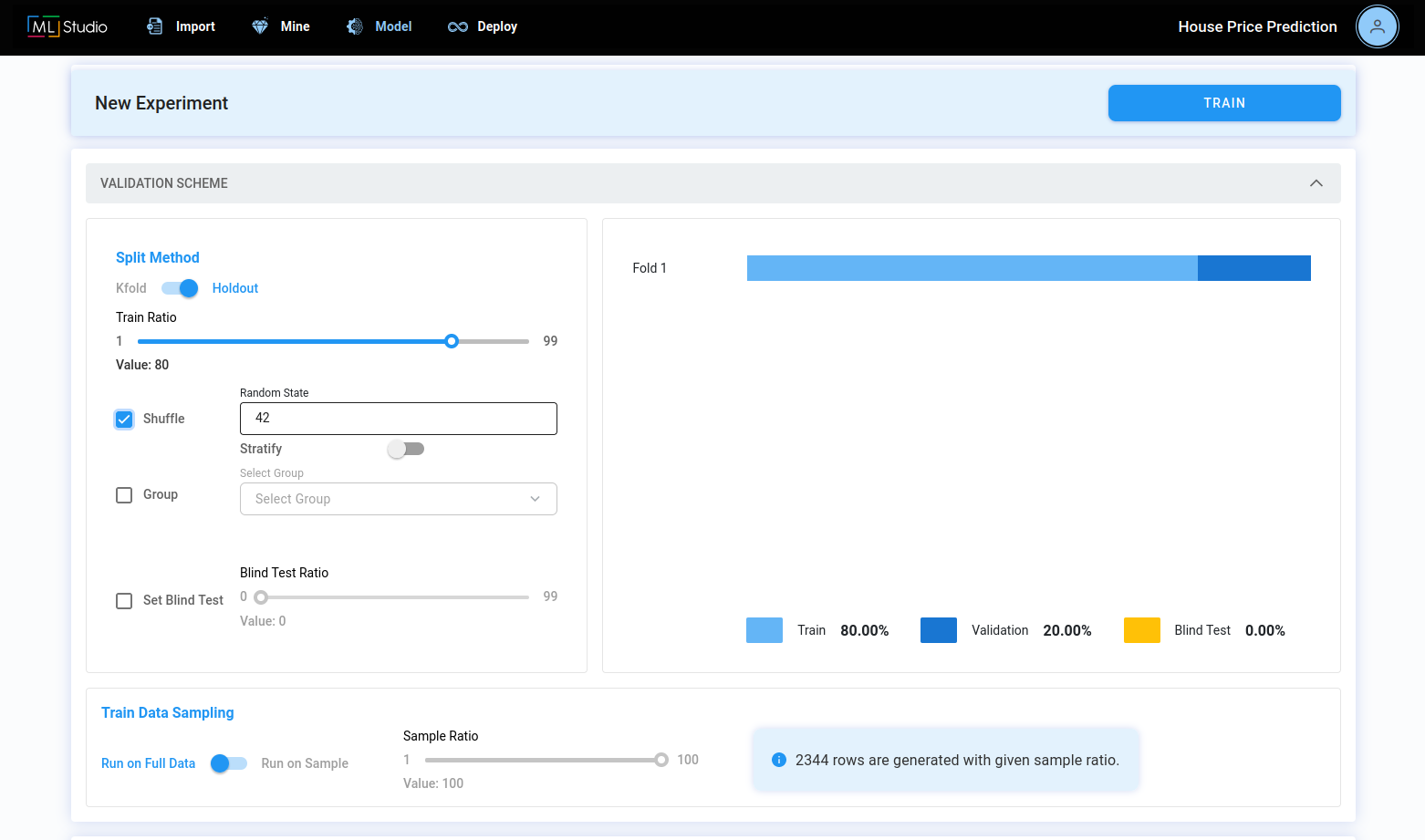Finally, LightGBM model is selected at the model selection stage. Octai uses state of the art optimization techniques for hyperparameter tuning so you don't have to worry about tuning hyperparameters of the LightGBM model. You can click "Train" button and watch your experiment's progress.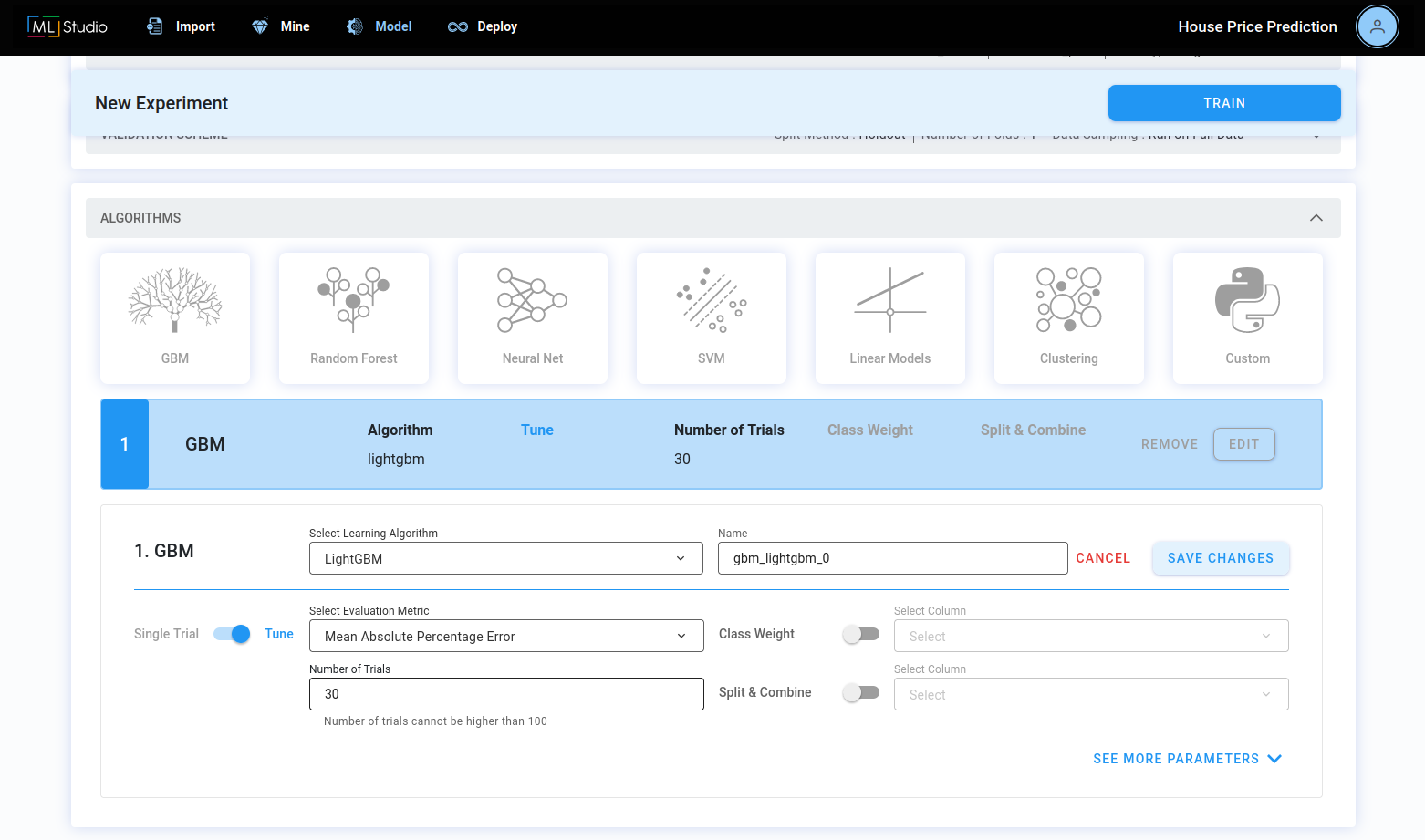When the experiment is finished, a champion model among trials is displayed. It is the model with the highest validation MAPE which is 8.4%.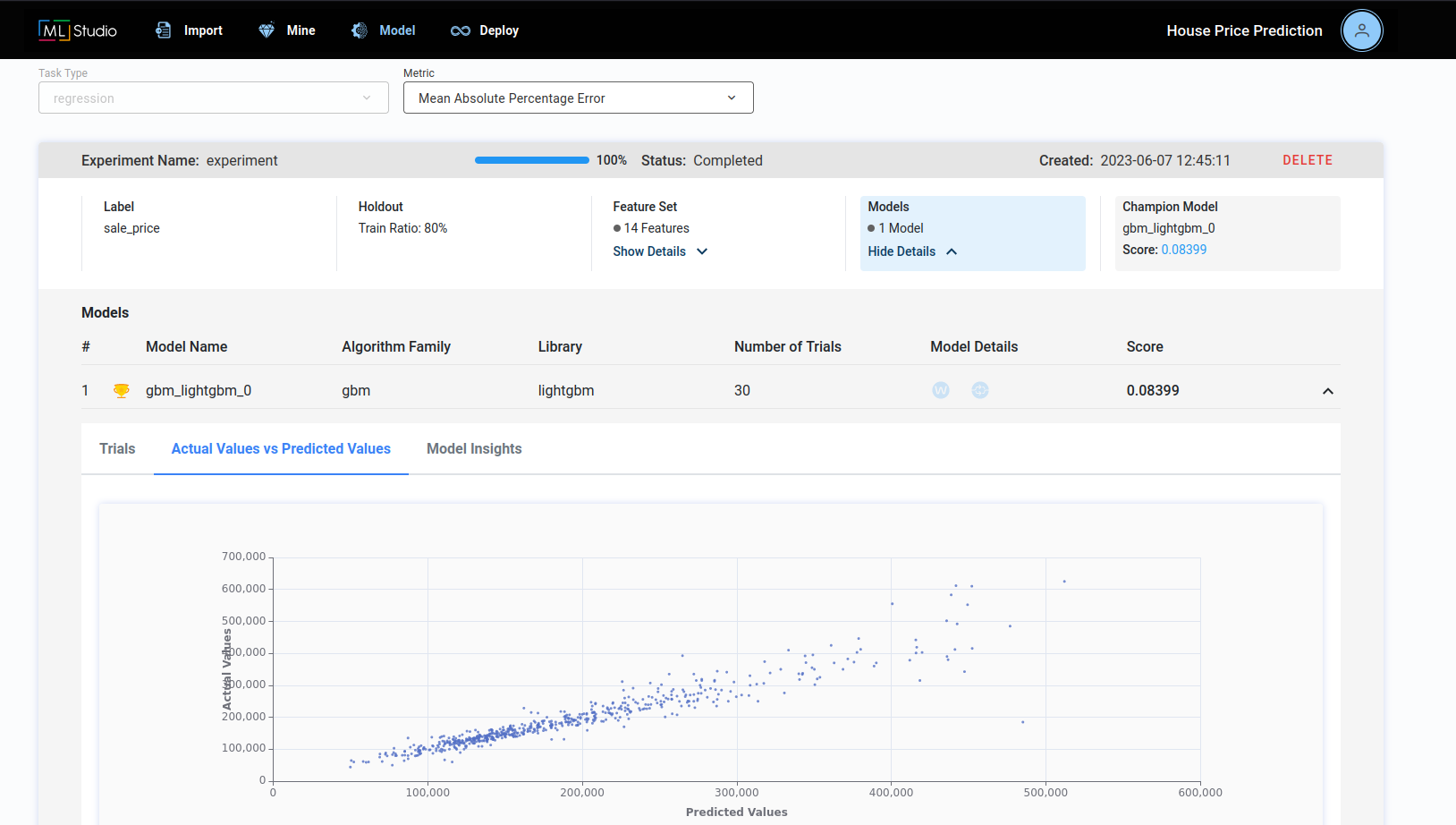To conclude, this would be the most profitable model to use for predicting price of your house because it is two times better than the baseline statistical approach. This model can be used by simply giving features of your house as inputs to the model. By doing that, the model will predict a price based on the given features.